# 2nd PUC Basic Maths Question Bank Chapter 11 Linear Programming Problems Ex 11.2

Students can Download Basic Maths Exercise 11.2 Questions and Answers, Notes Pdf, 2nd PUC Basic Maths Question Bank with Answers helps you to revise the complete Karnataka State Board Syllabus and score more marks in your examinations.

## Karnataka 2nd PUC Basic Maths Question Bank Chapter 11 Linear Programming Problems Ex 11.2

Part – A

2nd PUC Basic Maths Linear Programming Problems Ex 11.2 Five Marks Questions with Answers

Solve the following linear programming problems using graphical method

Question 1.
Maximize z = 60x + 15y
subject to x+y ≤ 50
3x + ≤ 90
x, y ≥ 0
Consider line x + y = 50
Put x = 0, y = 50, (0,50)
Put y = 0, x = 50 (50,0)
Consider the line 3x + y =- 90
Put x = 0, y = 90 (0,90)
Put y = 0, x = 30 (30,0)
Plot the above lines on the graph we get OABC is the solution region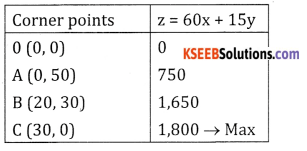≥ is maximum at c (30,0)
zmax = 1800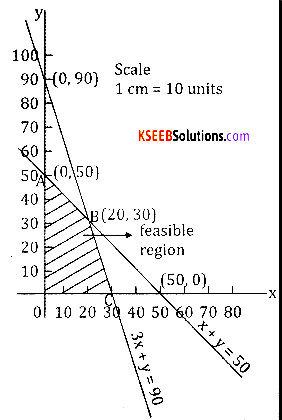Question 2.
Maximise z = 5x + 3y subject to
3x + 5y ≤ 15
5x + 2y ≤ 10
x ≥ 0,7 ≥ 0
Consider 3x + 5y = 15Plot the lines on the graph.
O ABC is the solution region.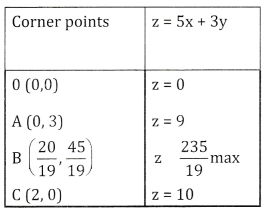X is maximum at B( $$\frac{20}{19}, \frac{45}{19}$$ )
zmax = $$\frac{235}{19}$$ = 12.37Question 3.
Minimise z = 3x + 5y
subject to x + 3y ≥ 3
X+ y ≥ 2
x, y: ≥ 0.
Consider x + 3y = 3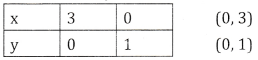0+0 ≯ 3
x + y = 2
False0+0 ≯  2
x + y = 2
False
∴ Shading is away from origin (0,0)z is minimum at B(1.5, 0.5)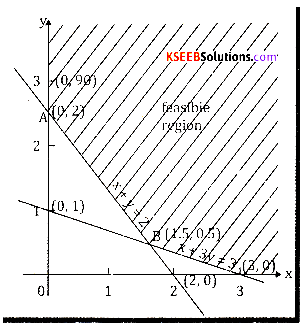Question 4.
Minimise z = x – 7y + 190 subject to
x + y ≤ 8
x ≤ 5, y ≤ 5, x + y ≥ 4, x ≥ 0, y ≥ 0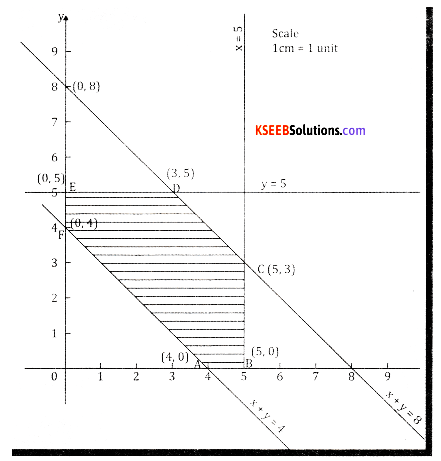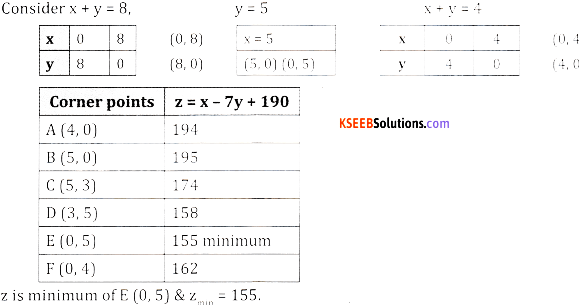z is minimum of E (0,5) & Zmin = 155.

Question 5.
Kellogg is a new cereal formed from a mixture of bran and rice that contains atleast 88 gm of protein and atleast 36 milligram of iron per kg knowing that bran contains 80 gm of protein and 40 milligram of iron per kilogram and that rice contains 100 gm of protein and 30 milligram of iron per kilogram, find the minimum cost of producing this new cereal if bran cost ₹ 5 kilogram and rice cost ₹ 4 per kilogram.
Let the units of Bran be x kg & Rice be y kg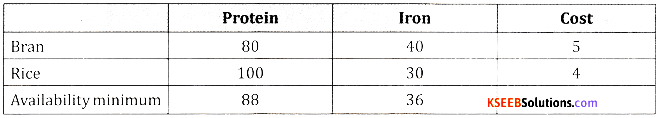Minimize Z = 5x + 4y subject to the constraints
80x + 100y ≥ 88
40x + 30 y ≥ 36
x, y ≥ 0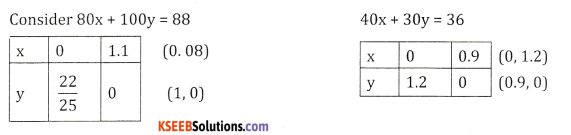Plot the times on the graph.z is minimum at B $$\left(\frac{3}{5}, \frac{2}{5}\right)$$
zmin = 4.6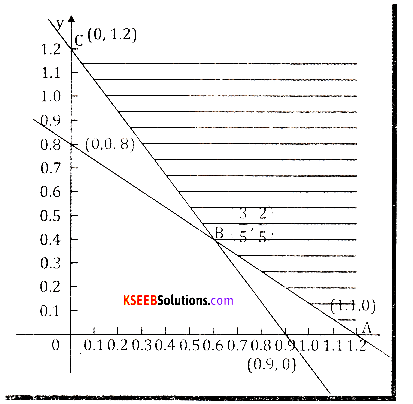Question 6.
A firm owned by Abhirami has to transport 1200 packages using large vans which can carry 200 packages each and small vans which can take 80 packages each. The cost for engaging each large van is ₹ 400 and each small van ₹ 200. Not more than ₹ 3000 is to be spent on the job and the number of large vans cannot exceed the number of small vans. Solve this L.P.P. graphically to find the minimum cost.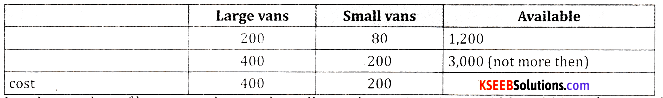Let the number of large vans be x and small vans be y minimize z = 400x + 200y subject to the constraints
200x + 80y ≥ 1,200
400x + 200y ≤ 3,000
x ≤ y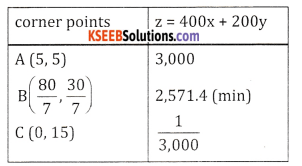z is min at B $$\left(\frac{30}{7}, \frac{30}{7}\right)$$
Zmin = 2,571.4.Question 7.
There are two types of fertilizers. F1 and F2 F1, consists of 10% nitrogen and 6% phosphoric acid and F, consists of 5% nitrogen and 10% phosphoric acid. After testing the soil conditions, a farmer Vaidhya finds that he needs atleast 14 kg of nitrogen and 14kg of phosphoric acid for the crop. If F1 costs ₹ 6 per kg and F2 costs ₹ 5 per kg, determine how much of each type of fertilizer should be used so that nutrient requirements are met at a minimum cost. Solve graphically.∴ z = 6x + 5y subject to the constraints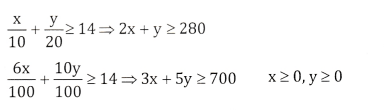Plot the lines in graphz is min at B(100,80) Zmin = 1,000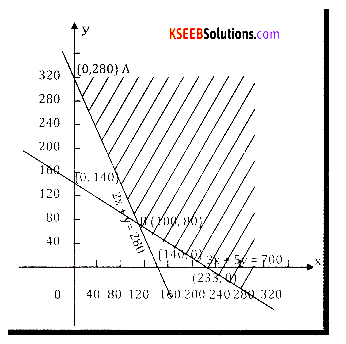Question 8.
A Television company owned by Priyanka and Bhavana operates two assembly lines, line land line II. Each of line is used to assemble the components of three types of television: colour standard and economy. The expected daily production on each line is as follows: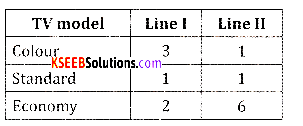The daily running costs fro two lines average ₹ 6000 for line I and ₹ 4000 for line II. It is given that company must produce atleast 24 colour, 16 standard and 48 economy TV sets for which an order is pending. Formulate L.P.P. and solve graphically determining the number of days the two lines should be run to meet the requirements.
Min Z = 6000x + 6000y subject to constraint
3x + y ≥ 24
x + y ≥ 16
2x + 6y ≥ 48
x, y ≥ 0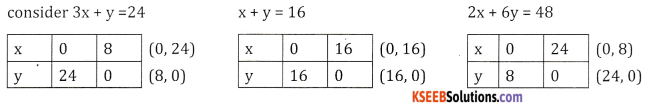plot the above lines on the graph.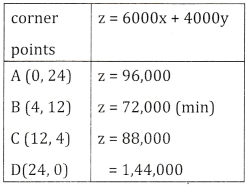z is minimum at B(4, 12)
z min = 72,000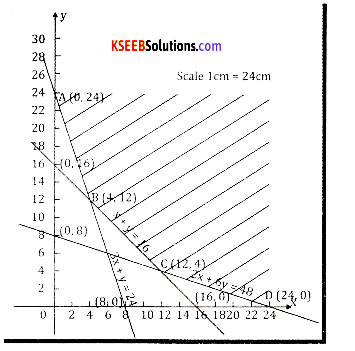Question 9.
Old hens can be brought at ₹ 2 each and young ones at ₹ 5 each. The old hens lay 3 eggs per week and the
young ones lay 5 eggs per week, each egg being worth 30 paise. A hen cost ₹ 1 per week to feed. Deepthi has only ₹ 80 to spend for hens. How many hens of each kind should Deepthi buy to give a profit of more than ₹ 6 per week assuming that Deepthi cannot house more than 20 hens. Solve graphically.
Max = 0.3 (3x + 5y) – (x + y) z = 0.5y – 0.1x subject to
x + y ≤ 20
2x + 5y ≤ 80
0.1 + 0.5y ≥ 6
x, y ≥ 0
Plot the lines on the graph and Solution is z is max” at (0,16) & zmax = 8

Question 10.
A company owned by Viswa Narayana concentrates on two grades of paper A and B, produced on a paper machine. Because of raw material restrictions, not more than 400 tonnes of grade A and 300 tonnes of grade B can be produced in a week. There are 160 production hours in a week. It requires 0,2 hour and 0.4 hour to produce one tone of the optimum product mix using the graphical method.
max z = 20x + 50 y subject to
0.2x + 0.4y ≤ 160
x ≤ 400
y ≤ 300 x, y ≥ 0
Plot the lines on the graph and solution is z is maximum at (200, 300) & zmax = 19,000

Question 11.
A company owned by Navya manufactures two types of cloth, using three different colours of wool. One yard length of type A cloth required 4 oz(once) of red wool, 5 oz of green wool, 3 oz (ounce) of yellow wool. One yard length of type B cloth requires 5 oz red wool, 2 oz of green wool and 8 oz of yellow wool. The wool available for manufacture is 1000 oz of red wool 1000 oz of green wool and 1200 oz of yellow wool. The manufactures can make a profit of ₹ 5 on one yard of type A cloth and ₹ 3 on one yard of type B cloth. Find the best combination of the quantities of type A and Type B cloth which gives him maximum profit by solving the L.P.P. by graphical method.